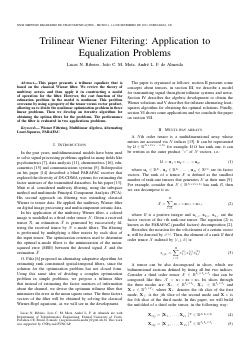##### Trilinear Wiener Filtering: Application to Equalization Problems

###### Abstract
This paper presents a trilinear equalizer that is based on the classical Wiener filter. We review the theory of multiway arrays and then apply it in constructing a model of operation for the filter. However, the cost function of the estimation problem in the model is nonlinear. This problem overcame by using a property of the tensor versus vector product, allowing us to divide the nonlinear optimization problem in three linear problems. Then we develop an iterative algorithm for obtaining the optima filters for the problems. The performance of the filter is evaluated in two applications problems.